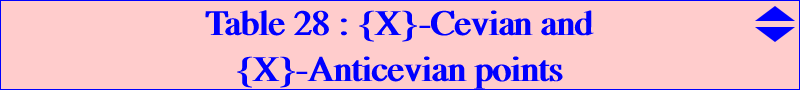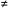A contribution by Wilson Stothers (8 November 1946 - 16 July 2009) This page is dedicated to his memory *** Preamble : extraversion, strong and weak geometric objects On this page, we use the algebraic form of extraversion as in Hyacinthos #7250. For a point X, {X} is the set of algebraic conjugates of X over the field generated by a^2, b^2, c^2. Then {X} is the set of algebraic extraversions of X. A function f(a,b,c) is a strong function if it is rational in a^2, b^2, c^2. An algebraic curve is a strong curve if it has an equation whose coefficients are strong functions. A point is a strong point if it has barycentric coordinates which are strong functions. Otherwise, X is a weak point. Equivalently, X is a strong point if the set {X} consists of X itself. Thus, X2, X3, X4, X5, X6 are strong, while X1, X7, X8, X9, X10 are weak, and each has four algebraic extraversions. Later, we shall meet points with up to ten extraversions. A line is strong if it has two strong points (or, equivalently, a strong tripole). A conic is strong if it has a strong perspector (or, equivalently, a strong centre). A pK cubic is strong if it has a strong pole and a strong pivot. We observe that, if X lies on a strong curve F, then all members of {X} are on F. We could equally define {X} as the intersection of all strong curves containing X. *** Definitions : A point P is an {X}-cevian point if P is a centre in {X} with respect to its Cevian triangle. A point P is an {X}-anticevian point if P is a centre in {X} with respect to its Anticevian triangle. See Table 45 for pedal equivalents. The most obvious example is that X2 is the only X2-Cevian, and the only X2-Anticevian point. We also know that (a) X4 is the only {X1}-Cevian point. (b) the members of {X1} are the X4-Anticevian points, (c) the members of {X7} are the X6-Cevian points. (d) X6 is the only {X7}-Anticevian point. Examples (a), (b) and (c), (d) are related by the trivial Proposition 1 : Y is an {X}-Cevian point if and only if X is a {Y}-Anticevian point. Notations : If X is any point, X' denotes the complement of the isotomic conjugate of X. In other words, X' is the CT-transform of X or simply X' = ctX. The inverse is the TA-transform X" = taX, the isotomic conjugate of the anticomplement of X. Examples (a), (d) and (b), (c) are special cases of the more interesting Proposition 2 : Y is an {X'}-Cevian point if and only if Y' is an {X}-Anticevian point. Proof : Note that the Cevian triangle of X and the Anticevian triangle of X' are homothetic so we can use the sidelengths of either in homogeneous formulas for centres. If a triangle centre X' is given by barycentrics f:g:h = f(u,v,w):f(v,w,u):f(w,u,v), where u, v, w are sidelengths, then X is given by 1/(f-g-h):1/(g-h-f):1/(h-f-g). The point Y = p:q:r with respect to ABC is q+r:r+p:p+q with respect to its Cevian triangle. Also, Y = p:q:r with respect to ABC is q+r-p:r+p-q:p+q-r with respect to its Anticevian triangle. Thus Y' is 1/p:1/q:1/r with respect to the Anticevian triangle of Y'. Now the conditions for Y to be an {X}-cevian point are equations f/(q+r)-g/(r+p) = 0, etc. The conditions for Y' to be an {X'}-Anticevian point are of the form p/(f-g-h)-q/(g-h-f) = 0. The two systems are patently equivalent. If a centre X is on a strong curve F, then we can define two loci: cF = { Y : Y is an {X}-Cevian point }, and aF = { Y : Y is an {X}-Anticevian point }. The condition that F is strong ensures that it contains all members of {X}. It also means that F can be defined using squares of sidelengths. Since the squares of the sidelengths of Cevian and Anticevian triangles can be computed easily, we can identify the curves cF and aF. For example, suppose that the cevian triangle of Y = x:y:z has sidelengths with squares P, Q, R, and that the coefficients of F are given in terms of a^2, b^2, c^2. Then we simply replace a^2, b^2, c^2 by P, Q, R and x, y, z by y+z, z+x, x+y in F(x,y,z) = 0 to get an equation for cF. Obviously aF is obtained similarly. *** A remarkable pencil of quintics Let m and n be two real numbers. Q[m,n] denotes the quintic m Q034 + n Q042. This pencil includes the Stothers Quintic Q012 = Q[-1,2] and other quintics as seen below. Also, Q[1,-1] is the union of the Kiepert Hyperbola and the sidelines. We have the result that the {X}-Cevian points for X on a Q[m,n] lie on another member of the pencil. Likewise for the {X}-Anticevian points. Proposition 3 : If F = Q[m,n], then cF = Q[3m+2n,-2(m+n)], and aF = Q[-2(m+n),2m+3n]. If F = Q012 = Q[-1,2] or Q059 = Q[2,-1], then F = cF = aF. Note that Q012 = Q[-1,2] and Q059 = Q[2,-1] contain all the associated Cevian and Anticevian points. This property is shared by the Euler-Morley Quintic Q003. These and other interesting F, cF, aF are given in the following table.cF : Locus of {X}-Cevians X on curve F aF : Locus of {X}-Anticevians Notes Lucas Cubic K007 Thomson Cubic K002 Sextic Q057 Note 1 Sextic Q058 Lucas Cubic K007 Thomson Cubic K002 Note 2 K117 Q027 K124X(370)-Quintic Q034 = Q[1,0] Kiepert Hyperbola = Q[1,-1] Quintic Q042 = Q[0,1]Quintic Q061 = Q[3,-2] Quintic Q034 = Q[1,0] Kiepert Hyperbola = Q[1,-1] Note 3 Kiepert Hyperbola = Q[1,-1] Quintic Q042 = Q[0,1] Quintic Q060 = Q[-2,3]Stothers Quintic Q012 = Q[-1,2] Stothers Quintic Q012 = Q[-1,2] Stothers Quintic Q012 = Q[-1,2]Quintic Q059 = Q[2,-1] Quintic Q059 = Q[2,-1] Quintic Q059 = Q[2,-1] Note 7 Euler-Morley Quintic Q003 Euler-Morley Quintic Q003 Euler-Morley Quintic Q003 Note 4 Yiu Quintic Q006 Circumcircle Diagonal Polar Circle Note 5 Anticomplement of Steiner Ellipse Steiner Ellipse Steiner InellipseNote 1 : It follows that the Lucas Cubic contains the {X4}-Cevians - the CCPC-points, there are 4 of these, the {X3}-Cevians - the central cyclocevian points, there are 7 of these, the {X9}-Cevians, there are 10 of these, the {X57}-Cevians, there are 10 of these, as well as the known cases the {X1}-Cevian, X4, the {X6}-Cevians, the 4 members of {X7}. Note 2 : From Note 1 and Proposition 2, we see that the Thomson Cubic contains the {X253}-Anticevians, and there are 4 of these, the {X69}-Anticevians, and there are 7 of these, the {X8}-Anticevians, and there are 10 of these, the {X189}-Anticevians, and there are 10 of these, as well as the known cases the {X7}-Anticevian, X6, the X4-Anticevians, the 4 members of {X1}. By looking at the Anticevians of points on the Euler line (a septic), we also have the X20-Anticevians, there are 7 of these. Note 3 : This shows that the Kiepert Hyperbola contains the four {X3}-Anticevian points. Note 4 : This shows that the Euler-Morley Quintic Q003 contains the {X4}-Cevians - the CCPC-points, there are 4 of these, the {X1}-Anticevians, there are 4 of these, as well as the {X4}-Anticevians, the 4 members of {X1}, the {X1}-Cevian, X4. It will also contain the {X357}-Cevian and Anticevian points. Note 5 : These two rows show that the seven {X99}-Cevians are intersections of the anticomplement of the Steiner Ellipse and the Yiu Quintic Q006 (see a figure there). the four {X99}-Anticevians are intersections of the Steiner Inellipse and the Polar Circle. The latter will be complex if ABC is acute-angled. Note 6 : The quintic Q[m,n] introduced above contains X1 if and only it is Q[0,1] = Q042 X2 or X4 if and only it is Q[1,-1] = union of Kiepert Hyperbola and sidelines X3 if and only it is Q[1,0] = Q034 X6 if and only it is Q[-1,2] = Q012, the Stothers Quintic.   Note 7 : The quintic Q[m,n] is circular if and only if m+2n = 0. This gives Q[2,-1] = Q059.   *** Properties and special cases Each member of the pencil contains A, B and C, X2, X13, X14, Ma, Mb, Mc, the vertices of the Medial Triangle. The medians meet the curve again at the vertices of a triangle homothetic with ABC whose A-vertex is -m : m+n : m+n. This homothety has center G, ratio -(2m+n)/(m+2n). Moreover If m+n0, then A, B, C are double points, with tangents the bisectors of the angles at A, B, C. If m+n = 0 - Q[1,-1] - then A, B, C are triple points since the quintic degenerates into the Kiepert hyperbola and the sidelines of ABC. X2 is singular if and only if m+2n =0 (Q[2,-1] = Q059 has a flex at X2) or 2m+n=0 (Q[1,-2] = Q012 has a node at X2). If m+2n0, then the tangent at X2 is X2X6. A distinct pair of members have 25 intersections, namely A, B, C, which count as 6 each, X2, which counts as two, X13, X14, Ma, Mb, Mc. Some special features Q012 = Q[-1,2] has infinite points those of the Steiner Ellipse and the Thomson Cubic, the asymptotes at the latter concur at X6 meets X2X6 at X2 (twice), X6 and on the Steiner Ellipse, the tangents at Ma, Mb, Mc concur at X6. Q059 = Q[2,-1] has infinite points those of the Circumcircle and the Medians, meets X2X6 at X2 (thrice) and on the Circumcircle. More generally, Q[m,n] contains the infinite points of the circum-conic with perspector Z if and only if Z lies on the quartic Q062 which contains X2 (Steiner ellipse), X6 (Circumcircle), X523 (Kiepert hyperbola). Q095 = Q[1,1] is another interesting quintic of the pencil passing through the Steiner point X(99).